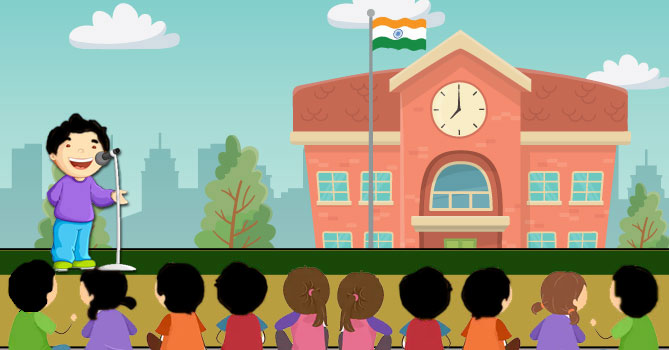# The sum of two numbers is 34. If 3 is subtracted from one number and 2 is added to another, the product of these two numbers becomes 260. Find the numbers.

Class 10th Maths Term 2, Question - The sum of two numbers is 34. If 3 is subtracted from one number and 2 is added to another, the product of these two numbers becomes 260. Find the numbers.